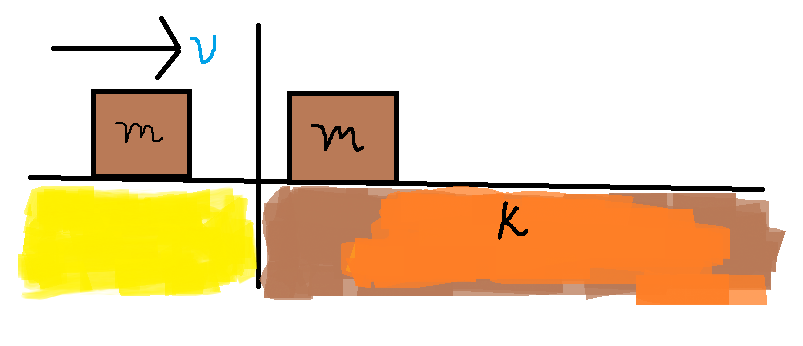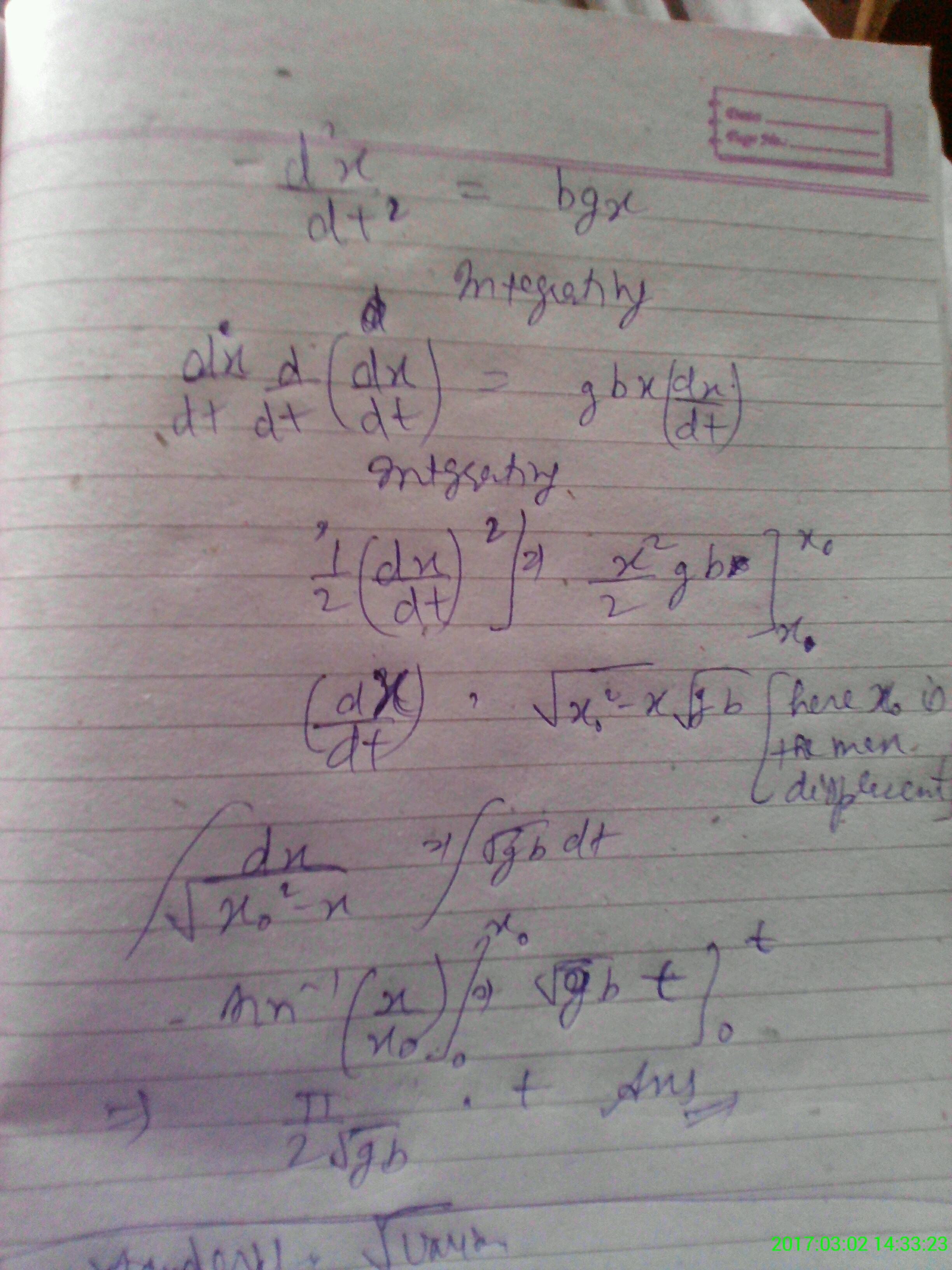# Evaluating time taken

I was trying to create a problem yesterday, something like this:The yellow region represents a smooth surface, while brown represents the rough one.

• A block of mass $m$ enters the rough region while initially traversing with a constant velocity $v$. We need to find the time taken by the block to come to rest.

The coefficient of friction between the block and the rough surface varies as $k=bx$, where $x$ is the distance traversed by the block on the rough surface (assuming the intersection of the axes looking lines as the origin) and $b$ is a positive constant.

As an initial approach, I tried to find out the stopping distance of the block, which goes like this.

$\displaystyle mv\frac { dv }{ dx } =-kmg$ or $mv\frac { dv }{ dx } =-bxmg$ as the only force acting on the block is friction.

$\displaystyle mvdv=-bmgxdx\\ m\int _{ v }^{ 0 }{ vdv= } -bmg\int _{ 0 }^{ { x }_{ 0 } }{ xdx }$

Giving

$\displaystyle \frac { { v }^{ 2 } }{ 2 } =\frac { bg{ { x }_{ 0 } }^{ 2 } }{ 2 } \\ \\ { x }_{ 0 }=\frac { v }{ \sqrt { bg } }$

But couldn't proceed on to evaluate time of motion. Please help me, thanks.

PS: Please rectify if there's any mistake in evaluating stopping distance.Note by Swapnil Das
4 years, 3 months ago

This discussion board is a place to discuss our Daily Challenges and the math and science related to those challenges. Explanations are more than just a solution — they should explain the steps and thinking strategies that you used to obtain the solution. Comments should further the discussion of math and science.

When posting on Brilliant:

• Use the emojis to react to an explanation, whether you're congratulating a job well done , or just really confused .
• Ask specific questions about the challenge or the steps in somebody's explanation. Well-posed questions can add a lot to the discussion, but posting "I don't understand!" doesn't help anyone.
• Try to contribute something new to the discussion, whether it is an extension, generalization or other idea related to the challenge.
• Stay on topic — we're all here to learn more about math and science, not to hear about your favorite get-rich-quick scheme or current world events.

MarkdownAppears as
*italics* or _italics_ italics
**bold** or __bold__ bold
- bulleted- list
• bulleted
• list
1. numbered2. list
1. numbered
2. list
Note: you must add a full line of space before and after lists for them to show up correctly
paragraph 1paragraph 2

paragraph 1

paragraph 2

[example link](https://brilliant.org)example link
> This is a quote
This is a quote
    # I indented these lines
# 4 spaces, and now they show
# up as a code block.

print "hello world"
# I indented these lines
# 4 spaces, and now they show
# up as a code block.

print "hello world"
MathAppears as
Remember to wrap math in $$ ... $$ or $ ... $ to ensure proper formatting.
2 \times 3 $2 \times 3$
2^{34} $2^{34}$
a_{i-1} $a_{i-1}$
\frac{2}{3} $\frac{2}{3}$
\sqrt{2} $\sqrt{2}$
\sum_{i=1}^3 $\sum_{i=1}^3$
\sin \theta $\sin \theta$
\boxed{123} $\boxed{123}$

## Comments

Sort by:

Top Newest

No its absolutely correct.Infact the time would be $t=π/(2√bg)$.Good to see you make your own prob.(☺☺)

- 4 years, 3 months ago

Log in to reply

Thanks :)

- 4 years, 3 months ago

Log in to reply- 4 years, 3 months ago

Log in to reply

Jai Ho :P

The mathematics was complicating for me, I believe, Thanks for the same. Had you encountered such type of question earlier?

- 4 years, 3 months ago

Log in to reply

not same but similar which i solved by other method ... first time using it :)

- 4 years, 3 months ago

Log in to reply

- 4 years, 3 months ago

Log in to reply

Can you tell where you are exactly stuck......BTW here we need to make use of $integral dx/√(a^2-x^2)=arcsin(x/a)+c$

- 4 years, 3 months ago

Log in to reply

clr or you want me to show it completely how to do ?

- 4 years, 3 months ago

Log in to reply

Show bhai please :P

- 4 years, 3 months ago

Log in to reply

×

Problem Loading...

Note Loading...

Set Loading...